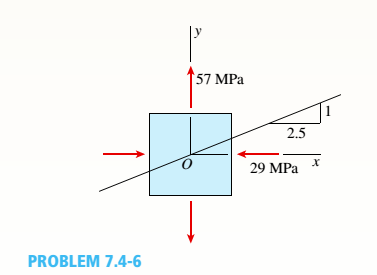# An element in biaxial stress is subjected to stresses a = -29 MPa and s y = 57 MPa. as shown in the figure. Using Mohr’s circle, determine the following. (a) The stresses acting on an element oriented at a slope of I on 2.5 (see figure). (b) The maximum shear stresses and associated norm al stresses. Show all results on sketches of properly oriented elements.### Mechanics of Materials (MindTap Co...

9th Edition
Barry J. Goodno + 1 other
Publisher: Cengage Learning
ISBN: 9781337093347
Chapter 7, Problem 7.4.6P
Textbook Problem
113 views

## An element in biaxial stress is subjected to stresses a = -29 MPa and sy = 57 MPa. as shown in the figure. Using Mohr’s circle, determine the following. (a) The stresses acting on an element oriented at a slope of I on 2.5 (see figure). (b) The maximum shear stresses and associated norm al stresses. Show all results on sketches of properly oriented elements.(a)

To determine

The stresses acting one an element oriented at a slope of 1on 2.5.

### Explanation of Solution

The stress acting on element along x-direction is 29MPaand along y-direction is 57MPa.

Write the expression to calculate the distance of center C from origin O along the horizontal direction.

OC=σx+σy2..…. (I)

Here the stress along the x-direction is σxand stress along the y-direction is and σy.

• Mark point A and B on the x-axis. OA represents stress acting on element along x-axis and OB represents stress acting on element along y-axis. Here shear stress is zero

Write the expression for the radius of Mohr’s circle.

R=|σx|+|σy|2        …… (II)

Here, radius of Mohr’s circle is R.

Write the expression for orientation of element.

θ=tan1(yx)        ……. (III)

Here, slope of the oriented element is θ, and intercept at x-axis is yand intercept at y-axis is x.

Write the expression for stress along center point.

σC=σx+R        …… (IV)

Here, stress along center point is σC.

Write the expression for minimum principle stress.

σx1=σCRcos(2θ)        …… (V)

Here, minimum principle stress is σx1.

Write the expression for maximum principle stress.

σx2=σC+Rcos(2θ)        …… (VI)

Here, maximum principle stress is σx2.

Write the expression for shear stress on oriented plane.

τx1y1=Rsin(2θ)        …… (VII)

Here, principal shear stress is τx1y1

Calculation:

Substitute 29MPafor σxand 57Mpafor σyin Equation (I).

OC=29MPa+57MPa2=28MPa2=14MPa

Substitute 29MPafor σxand 57Mpafor σyin Equation (II).

R=|29MPa|+|57MPa|2=(86MPa2)=43MPa

Substitute 1unitfor yand 2.5unitsfor xin equation (III)

• ### Still sussing out bartleby?

Check out a sample textbook solution.

See a sample solution

#### The Solution to Your Study Problems

Bartleby provides explanations to thousands of textbook problems written by our experts, many with advanced degrees!

Get Started

Find more solutions based on key concepts
List five drill press safety guidelines.

Precision Machining Technology (MindTap Course List)

What value does an automated asset inventory system have during risk identification?

Principles of Information Security (MindTap Course List)

What are the basic characteristics of a NoSQL database?

Database Systems: Design, Implementation, & Management

How many records does the file contain? How many fields are there per record?

Database Systems: Design, Implementation, & Management

What is the process that engineering managers use to ensure that a project is completed on time and within budg...

Engineering Fundamentals: An Introduction to Engineering (MindTap Course List)

Why would a manager prefer a decision tree instead of a decision table?

Systems Analysis and Design (Shelly Cashman Series) (MindTap Course List)

What is benchmarking?

Management Of Information Security

Many programs today use forms on a webpage as their front end. (505)

Enhanced Discovering Computers 2017 (Shelly Cashman Series) (MindTap Course List)

What is the maximum width of the cover pass? Why?

Welding: Principles and Applications (MindTap Course List)

If your motherboard supports ECC DDR3 memory, can you substitute non-ECC DDR3 memory?

A+ Guide to Hardware (Standalone Book) (MindTap Course List)